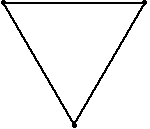# Chasing Turtles Problem

jpas
This is supost to be a classic. I solved it but my answer isn´t right and I can´t find out why. Hope you guys can help.

Imagine an equilateral triangle with one turtle on each side of lenth L. All turtles move with velocity V. Turtle 1 always directs its motion to turtle 2, turtle 2 to turtle 3, and turtle 3 to turtle 1, again. Calculate the time the turtles take to meet.

The movement will be something like this.Relative to turtle 2, turtle 1 will be in uniform rectilinear motion and its velocity will be:

$$\vec V_{12}=\vec v_1 - \vec v_2$$

$$\vec v_1$$ and $$\vec -v_2$$ make an angle of 60º degrees. So,

$$V_{12}= \frac{V}{\sqrt{3}}$$

Relative to turtle 2, turtle 1 will only travel the distance that separates it from the center of the triangle, which is $$\frac{L}{3}$$

So, $$t= \frac{v_{12}}{d} = \frac{L}{3V}$$

P.S.: I guess some of you might have already solved this problem. If not, I recommend it, though I got it wrong.

Last edited by a moderator:

Bob S
You have an equilateral triangle with side length L. The velocity of each turtle is v. The triangle defined by the 3 points (turtles) is always at any time t an equilateral triangle. Because the distance d between the turtles (the side of the equilateral triangle) at time t is

d=L-vt,

the turtles meet when

L-vt = 0

Bob S

jpas

Because the distance d between the turtles (the side of the equilateral triangle) at time t is

d=L-vt,

This isn´t true. How did you arrive to this equation?

The real time the turtles take is $$\frac{2L}{3V}$$ , 1.5 times what you got.

Does anyone have other sugestions? I advise you, if you´ve got nothing to do, this is a fun problem!

Bob S
You are correct. The easiest way to get the solution is to use radial coordinates. The direct distance from the apex to the center is (from plane geometry)

R = L/√3

For a radial step dr, the turtle must always travel a distance 2·v·dt/√3

So to travel a radial distance R=L/√3,

v·t = (L/√3)(2/√3) = 2L/3

t=2L/3v

Bob S

jpas
Hi Bob, thanks for the reply.

R = L/√3

Right. On my orginal post I had a typo. I meant this.

For a radial step dr, the turtle must always travel a distance 2·v·dt/√3

Can you explain this step? What is dr and how did you calculate it?

Bob S
For a radial step dr, the turtle must always travel a distance 2·v·dt/√3.
Can you explain this step? What is dr and how did you calculate it?
A turtle step dx = v·dt is always at a 30-degree angle to the radial direction dr from the apex of the equilateral triangle (between the 3 turtles) to the center, because the radial direction always bisects the 60-degree angle of the equilateral triangle (even as the turtles move and the equilateral triangle rotates), and the instantaneous direction of v·dt is always parallel to one of the sides of the equilateral triangle. Using plane geometry, the longer side of a 30-60-90 degree triangle is equal to √3/2 times the hypotenuse. The turtle step v·dt is along the hypotenuse and the radial step projection dr is along the longer side.

So a radial step dr = (√3)·v·dt/2

Bob S